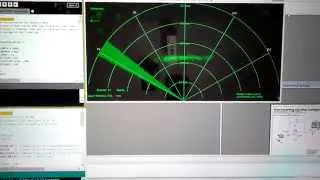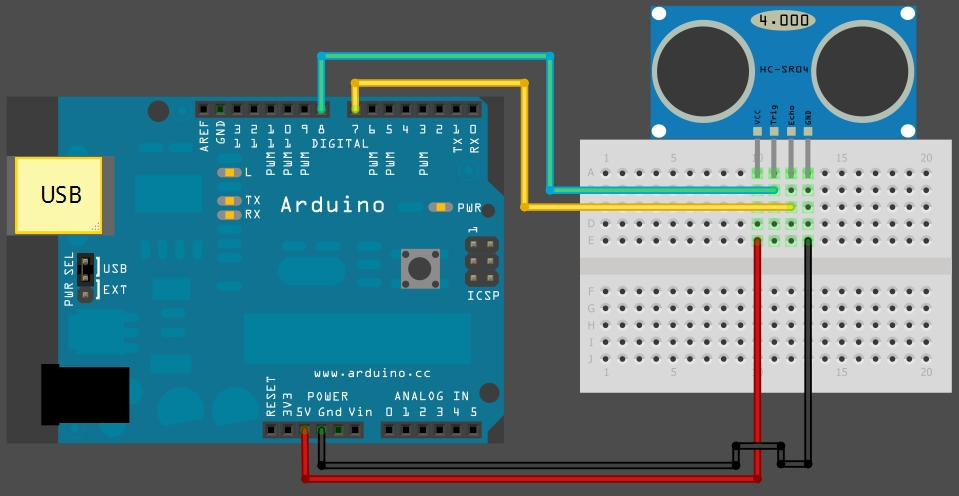Project tutorialUse HC-SR04 on Arduino with Processing

• 140,328 views
• 247 respects

## Components and supplies

Arduino Code:

Sends sensor readings for every degree moved by the servo values sent to serial port to be picked up by Processing

Processing Code:

Maps out an area of what the HC-SR04 sees from a top down view. Takes and displays 2 readings, one left to right and one right to left. Displays an average of the 2 readings

Any questions are welcome!

Github: https://github.com/faweiz

Portfolium: https://portfolium.com/faweiz

## Code

##### Untitled fileArduino
```/*
Sends sensor readings for every degree moved by the servo
values sent to serial port to be picked up by Processing
*/

#include <NewPing.h>
#include <Servo.h>

#define TRIGGER_PIN  2   // Arduino pin 2 tied to trigger pin on the ultrasonic sensor.
#define ECHO_PIN     3   // Arduino pin 3 tied to echo pin on the ultrasonic sensor.
#define MAX_DISTANCE 150 // Maximum distance we want to ping for (in centimeters). Maximum sensor distance is rated at 400-500cm.
#define SERVO_PWM_PIN 9 //set servo to Arduino's pin 9

// means -angle .. angle
#define ANGLE_BOUNDS 80
#define ANGLE_STEP 1

int angle = 0;

// direction of servo movement
// -1 = back, 1 = forward
int dir = 1;

Servo myservo;
NewPing sonar(TRIGGER_PIN, ECHO_PIN, MAX_DISTANCE);

void setup() {
Serial.begin(9600); // initialize the serial port:
myservo.attach(SERVO_PWM_PIN); //set servo to Arduino's pin 9
}

void loop() {

delay(50);
// we must renormalize to positive values, because angle is from -ANGLE_BOUNDS .. ANGLE_BOUNDS
// and servo value must be positive
myservo.write(angle + ANGLE_BOUNDS);

// read distance from sensor and send to serial
getDistanceAndSend2Serial(angle);

// calculate angle
if (angle >= ANGLE_BOUNDS || angle <= -ANGLE_BOUNDS) {
dir = -dir;
}
angle += (dir * ANGLE_STEP);
}

int getDistanceAndSend2Serial(int angle) {
int cm = sonar.ping_cm();
Serial.print(angle, DEC);
Serial.print(",");
Serial.println(cm, DEC);

}
```

## Schematics#### Security Access Using RFID Reader

• 472,842 views
• 499 respects

#### Ultrasonic Map-Maker using an Arduino Yun

Project tutorial by Satyavrat Wagle

• 84,928 views
• 195 respects

#### Windows 10 IoT Core: UltraSonic Distance Mapper

Project showcase by Anurag S. Vasanwala

• 65,230 views
• 173 respects

#### Motion Following Motorized Camera Base

Project tutorial by Lindsay Fox

• 152,012 views
• 388 respects

#### Arduino Bluetooth Basic Tutorial

• 869,677 views